Courses

# Test: Electronic Devices - 5

## 10 Questions MCQ Test RRB JE for Electronics & Communication Engineering | Test: Electronic Devices - 5

Description
This mock test of Test: Electronic Devices - 5 for Electronics and Communication Engineering (ECE) helps you for every Electronics and Communication Engineering (ECE) entrance exam. This contains 10 Multiple Choice Questions for Electronics and Communication Engineering (ECE) Test: Electronic Devices - 5 (mcq) to study with solutions a complete question bank. The solved questions answers in this Test: Electronic Devices - 5 quiz give you a good mix of easy questions and tough questions. Electronics and Communication Engineering (ECE) students definitely take this Test: Electronic Devices - 5 exercise for a better result in the exam. You can find other Test: Electronic Devices - 5 extra questions, long questions & short questions for Electronics and Communication Engineering (ECE) on EduRev as well by searching above.
QUESTION: 1

### The figure shows the VTC characteristics of an NMOS inverter with three varying resistive loads (R).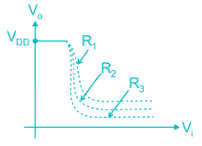The correct statement is:

Solution: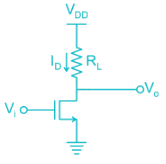• As Vi is increased voltage drop across RL increases and V0 decreases.
• The sharpness of the transition region increases with the increasing load resistance.
• Hence R3 > R2 > R1.
*Answer can only contain numeric values
QUESTION: 2

### Assume that the zero for electrostatic potential is in the semiconductor bulk at large x and that there is no metal semiconductor work function difference. The relative dielectric constant for the oxide is ϵr = 11.8. If the intrinsic concentration is 1010/cm3. The doping density ND is _____ × 1017/cm3 (KT = 0.026 V)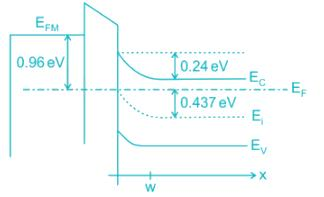Solution:

The doping density is given by: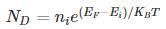= 1010 e(0.437/0.026)

= 1.99 × 1017

QUESTION: 3

### The figure shows MOS capacitor variation with applied gate voltage for n-type body/substrate.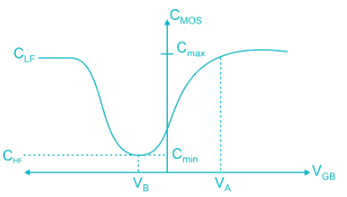The flat band voltage is:

Solution: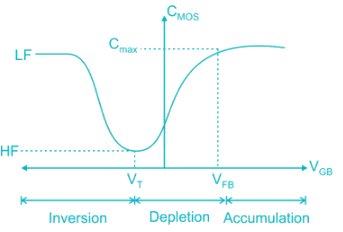• Flat band voltage is the voltage where there is no charge present in oxide or oxide-semiconductor interface. i.e. No thickness of channel
• Cox is constant and decreased subsequently
Note: Here, VG is negative (for PMOS)
• Hence, voltage is decreased from positive (VFB) to negative.
*Answer can only contain numeric values
QUESTION: 4

The insulator capacitance Ci of an ideal MOS capacitor with 10-nm gate oxide (εr = 3.9) on p-type Si with Na = 1016 cm-3 is ________ × 10-7 F/cm2

Solution:

The insulator capacitance is the capacitance under strong accumulation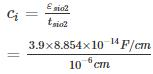= 3.453 × 10-7 F/cm2

QUESTION: 5

Which of the following curves represents the correct C – V characteristics of an NMOS transistor having an oxide layer thickness of 10 nm and a maximum depletion thickness of 100 nm. [Assume ϵs = permittivity of semiconductor, ϵox = permittivity of oxide and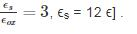Solution:

Given, tox = 10 nm, d = 100 nm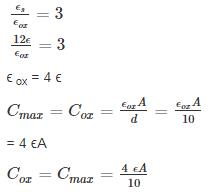Cmin = series combination of Cox and Cdep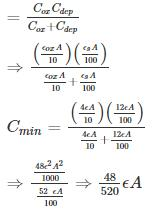= 0.09 ϵA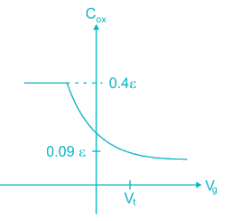QUESTION: 6

The mobility of hole is 0.4 times the mobility of electron. What must be the ratio of width of n-channel to p-channel MOSFET if they are to have equal drain currents when operated in saturation mode with same magnitude of overdrive voltage:

Solution:

ID-n = ID-p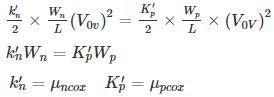μn Wn = μp Wp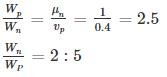QUESTION: 7

The circuit shown uses an NMOS transistor to implement a current source. For the transistor VTN = 1V and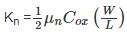=12.5 uA/V2. The required value of VGS to get IDC = 25 μA and corresponding compliance voltage is: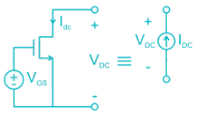Solution: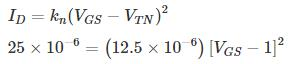2 = (VGS - 1)2‑
VGS = √2 + 1 = 2.414 V

To function as a current source, the transistor must be in saturation or VDS > VDS (sat).

VDS (sat) = VGS - VTN

= 2.414 – 1

= 1.414.

Hence compliance voltage = 1.414 V.
Compliance voltage is the minimum voltage required to achieve the desired performance.

*Answer can only contain numeric values
QUESTION: 8

The output resistance R0 of the NMOS circuit if ID = 0.5 mA, λ = 0.02 V-1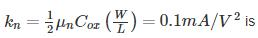_____ kilo ohms.Solution:

The small signal model with a test voltage Vx is shown.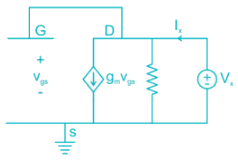The output resistance is given by: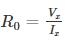From the circuit, Vgs = Vx

Applying KCL: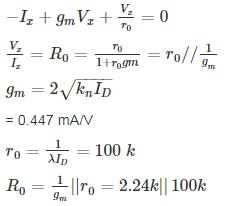QUESTION: 9

For a MOSFET with gate plate area 0.5 × 10-2 cm2 and oxide layer thickness 80 nm, the value of MOS capacitance and its break down voltage are: (assume relative di-electric constant of sio2, ϵr = 4 and ϵ0 = 8.854 × 10-14 F/cm and dielectric strength of sio2 film is 5 × 106 V/cm)

Solution:

Given gate plate area, which is also MOS capacitor area A = 0.5 × 10-2cm2

Oxide layer thickness tox = 80 nm

The value of MOS capacitance: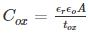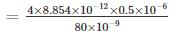= 0.22 μF

Dielectric breakdown happens of field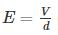greater than dielectric strength (5 × 106 V/cm)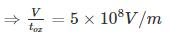V = 5 × 108 × 80 × 10-9 V = 40V

*Answer can only contain numeric values
QUESTION: 10

In the circuit shown in Figure, Transistors are characterized by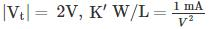and λ = 0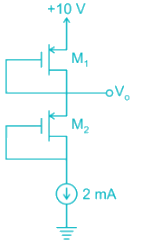The output voltage V0 is _______V

Solution:

Gate is connected to drain Both Transistor are in Saturation.

For M1 Transistor: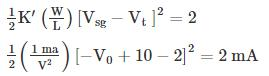⇒ (V - 8)2 = 4
⇒ V - 8 = ± 2

(i) Taking +ve, V = 8 + 2 = 10 V (Not Possible)
(ii) Taking -ve, V = 8 - 2 = 6 V